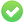# Rounding to next 100,000, or some such

I have a proposed budget with three different scenarios, called “A,” “B” and of course, “C.” Each has different budget amounts in certain categories, dependent on the scenario. I insert the applicable budget amount using the Data Validation Tool along with an IF statement, so that `=IF(A1="A","A Shows",IF(A1="B","Now B Shows",IF(A1="C","And Finally C Shows")))` (an example) works to give me the desired value in the correct box.

However, I want to make a calculation that rounds up my totals in a way that is applicable to each scenario, and which requires that I round up to three different larger increments, 100,000, 200,0000 and 300,000. I do not want to merely add those amounts, I want the totals rounded up to the next increment of those.

Example, if A is \$932, then it might round up to \$100,000, while if B were \$273,000, it would round up to \$400,000 and C if it was \$675,000 would round up to \$678,000.

I can’t find anything other than someone who wanted to round down to the next 100, but I couldn’t get that to work.

Anyone have any ideas?

For the 1st case, that would be: `=ROUNDUP(A1/100000;)*100000` with A1 being the cell to be rounded.

For the 2nd, that would be: `=ROUNDUP(A1/400000;)*400000`

The 3rd condition is rather strange. But I guess you got the idea.

To show the community your question has been answered, please tag the best answer (), and/or vote for any helpful answer. Else, please edit your question to add information (answers are for solutions only).

Or better for first case:

``=ROUNDUP(A1;-5)``

I like the solution, but I am losing it in my mechanics. Here is what I have, hopefully you can see why I added the subtraction at the end. The formula drops everything after the divisor sign, which I presume is because of misplaced parentheses or some such, but I have counted and do not see my error. Here is what I have developed for my use case.

``````=ROUNDUP(SUM(SUM(D44:D65))/(IF(\$K\$10="Arena_Cost",500000,IF(\$K\$10="Box_Truck_Cost",125000,IF(\$K\$10="Corral_Cost",250000)));)*IF(\$K\$10="Arena_Cost",500000,IF(\$K\$10="Box_Truck_Cost",125000,IF(\$K\$10="Corral_Cost",250000))))
``````

Any idea what I am doing wrong?

Separators are wrong or missing. Use semi colons instead of comas.

``````=ROUNDUP(SUM(SUM(D44:D65))/(IF(\$K\$10="Arena_Cost";500000;IF(\$K\$10="Box_Truck_Cost";125000;IF(\$K\$10="Corral_Cost";0,25)));)*IF(\$K\$10="Arena_Cost";500000;IF(\$K\$10="Box_Truck_Cost";125000;IF(\$K\$10="Corral_Cost";0,25))))
``````

Cannot check the end result (division by 0) but it should work with your data.

The `Ceiling Function` is getting me to where I need to be, and seems that it may be able to handle all my use cases.

A working solution for my use is `=CEILING(D95, 100000)+(IF(\$K\$10="Arena_Cost",500000,IF(\$K\$10="Box_Truck_Cost",250000,IF(\$K\$10="Corral_Cost",250000))))`